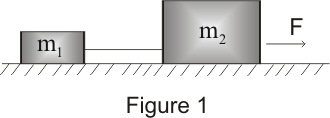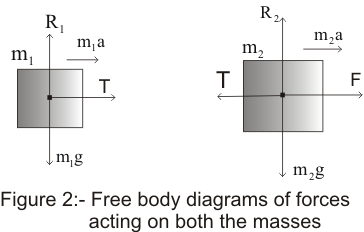# How to Apply Newton's laws of motion

### (7) Applying Newton's law of motion

• Newton's law of motion ,we studied in earlier topics are the foundation of mechanics and now we look forward to solve problems in mechanics
• In general, we deal with mechanical systems consisting of different objects exerting force on each other
• While solving a problem choose any part of the assembly and apply the laws of motion to that part including all the forces on the chosen part of the assembly due to remaining parts of the assembly
• Following steps can be followed while solving the problems in mechanics
2) Draw a schematic diagram showing parts of the assembly for example it may be a single particle or two blocks connected to string going over pulley etc
3) Identify the object of prime interest and make a list of all the forces acting on the concerned object due to all other objects of the assembly and exclude the force applied by the object of prime interest on the other parts of the assembly
4) Indicate the forces acting on the concerned object with arrow and Label each force for example tension on the object under consideration can be labelled by letter T
5) Draw a free body diagram of the object of interest based on the labelled picture.Free body diagram for the object under consideration shows all the forces exerted on this object by the other bodies.Do not forget to consider weight W=mg of the body while labelling the forces acting on the body
6) If additional objects are involved draw separate free body diagram for them also
7)Resolve the rectangular components of all the forces acting on the body
8) Write Newton second law of equation for the body and solve them to find out the unknown quantities
9) Do not forget to employ Newton's third law of motion for action reaction pair which results in null resultant force

• Following solved example would clearly illustrate how to apply Newton's laws of motion following the above given procedure

Solved Example :
Question:
A horizontal forces of magnitude 500N pulls two blocks of masses m1=10 kg and m2=20 kg which are connected by the light inextensible string and lying on the horizontal frictionless surface.Find the tension in the strings and acceleration of each mass when forces is applied on mass
m2? Solution:
Given that force is applied on the block m2 as shown in the figure belowLet T be the tension in the string and a be the acceleration of each mass .Now we will draw free body diagrams for each massesWeights of the blocks m1g and m2g are balanced by their normal reaction R1 and R2 respectively.The equations of motion of the two massed are found using Newton's second law of motion
m1a=T ...............................(1)
m2a=F-T ............................(2)

Dividing 1 by 2
we get
T=m1F/(m1+m2)
Substituting the given values
T=166.7 N
Using value of T in equation 1 ,we find
a=16.67 m/s2

Above sample problem shows how to solve a typical mechanics problem.Similarly by adopting given procedure we can solve other such problems
Watch this tutorial on how to solve Newton law of motion problem